Next: Input digits required Up: Limits within [-1,1] Previous: Limits within [-1,1]

### Determining output digits

Suppose we have inputs (x,y,z) where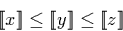We examine the first digits of x and z, and use simple analysis of the range of possible reals represented, as described in section 3.6.2 to determine whether or not we can determine digits of a stream representing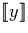using only the known digits of x and z.

Having examined a some finite number of digits from x and z, we will encounter on of three qualitatively distinct cases; either we can say that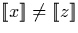, or we can find a digit or digits whose range encloses all possible values of, or we cannot find such a digit but cannot say that. In the first case it is safe to cease examination of x and z and use y to return the remainder of the result. In the second and third cases we must continue to examine x and z, generating digits where possible, until we can say for certain that.

Suppose the ranges r = [rmin, rmax] and s = [smin,smax] contain the lower and upper bounds x and z of y. There are a number of possible situations that may arise. Figure 5.1 illustrates with some examples.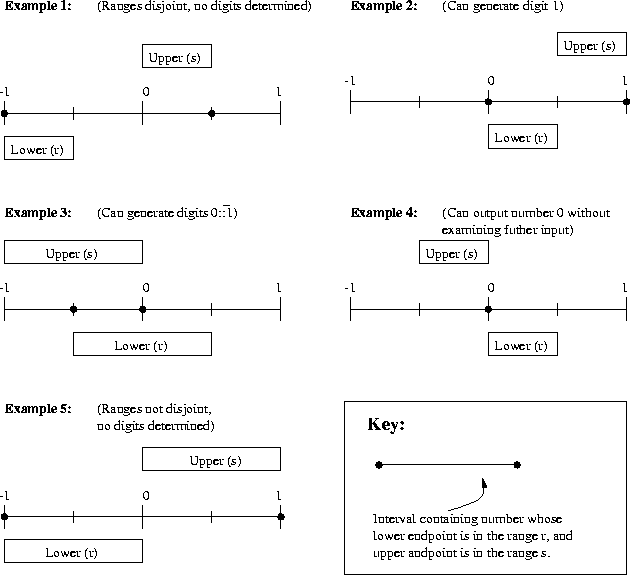Case 1:
The lower and upper bounds are too far apart to determine a digit, and ranges are disjoint. Therefore lower and upper bounds not equal and we can safely return y.

Example 1 illustrates such a situation.

Case 2:
We can find a digit such whose range encloses [rmin, smax]. Output this digit and examine the inputs further.

In example 2, we can deduce that the range of y is [0,1], so we can generate the digit 1 as the first digit of y. Similarly in example 3, the range of y must be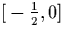, so we can generate the digits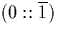(or equivalently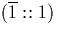).

Example 4 is an interesting possibility which would be handled by here. We can see that the least possible value of the lower bound rmin is equal to the greatest possible value of the upper bound smax. We can deduce that this is the only possible value for y and output it directly without examining further inputs or intervals.

Case 3:
We cannot find a digit such whose range encloses [rmin, smax], but we cannot say for certain that the lower and upper end-points are not equal. Therefore we must examine more digits of the end-points.

Example 5 falls into this category. Suppose the numeraland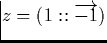, we can see that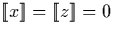. If this were the case, we would have to output the number zero (eg. the numeral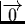) without examining y or any further intervals by the condition stated in section 5.1. We must therefore examine further digits of x and z until either we can ascertain that they are both equal, or we can generate a digit as in examples 2, 3, or 4.Next: Input digits required Up: Limits within [-1,1] Previous: Limits within [-1,1]
Martin Escardo
5/11/2000